# Fibonacci Retracement 101

| |

Fibonacci, seems like some magical tool that we traders have in our arsenal right? If you have some experience in trading then you know what I am talking about.

Did you know that the Fibonacci retracement tool that is available on the trading platforms is derived out of some naturally occurring phenomena? If you find this exciting enough then this blog post is for you.

In this blog post, I will discuss everything you need to know about Fibonacci and the Fibonacci retracement tool that you can use in trading.

Stick around till the end for a strategy involving the Fibonacci tool, using which I have managed to catch good chunks of pips and profited approximately \$42,000.

### Contents

What is Fibonacci?

How is Fibonacci relevant in trading?

What is Fibonacci retracement?

Fibonacci retracement strategy

## What is Fibonacci?Leonardo Bonacci, also known as Leonardo Fibonacci, wrote a book titled Liber Abaci, “The book of calculation”, in the year 1202.

It was through this book that he introduced the concept of Fibonacci numbers.

This is a mathematical concept, which can be found to be present in almost all walks of life. The Fibonacci numbers form a sequence known as the Fibonacci sequence.

In the Fibonacci sequence, each number is a sum of the previous two numbers and the sequence continues infinitely. The sequence starts with numbers 0 and 1.

Fibonacci sequence – 0,1,1,2,3,5,8,13,21,34,55,89,144,233, etc.

This series is not any random series created by a person. It is an intriguing one because every number in this Fibonacci sequence is exactly 1.618 times larger than the previous number in the sequence and every number is 0.618 times smaller than the number after it. This is how we get the 61.8% Fibonacci ratio.

When a number in this sequence is divided by a number in the sequence two spots away, we get a 38.2% ratio and when it is divided by a number located three spots on the right, then we get the 23.6% ratio.

For instance, 3 divided 5 will give us 0.6, 3 divided by 8 results 0.375, 3 divided by 13 is 0.23, which is 61.8%, 38.2% and 23.6% approximately respectively.

The ratio of 1.618% is said to be a golden ratio and is called Phi. As I mentioned already that the Fibonacci can be found to be present in all walks of life, the golden ratio can be found in the human body, nature, formation of stars, and the galaxy everywhere.

## How is Fibonacci relevant in trading?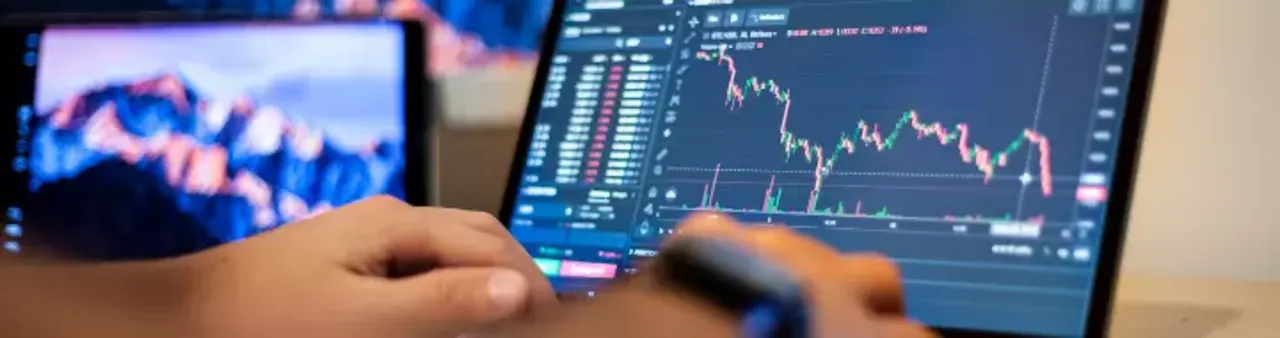The recurrence of this mathematical concept in such unrelated things does make it intriguing. It isn’t a random feat and there is no denying the fact that whatever it is, it does have some value.

There is a huge section of traders that use the Fibonacci ratios, 61.8%, 38.2%, and 23.6% to take trade decisions. Fibonacci analysis in the financial market has seen a great rise.

Traders look to apply this concept on the price charts of the various financial assets that are available to trade, they plot these ratios on the charts and look to forecast price movements around these levels.

The followers of this method of analysis argue that this phenomenon in nature works in the markets too. They look to use this in tandem with other tools and methods and take a holistic trade decision.

Like any other type of analysis, Fibonacci does have its fair share of critics. These sections of traders argue that the Fibonacci ratios are actually of no significance in the market and are a self-fulfilling prophecy.

Two sides to the coin, you have to decide which side to take. I have used this method, tested it out, and have earned decent profits and I will discuss the exact strategy I used. So stay attentive and read till the end.

## What is Fibonacci retracement?The Fibonacci retracement is a technical analysis tool that is a widely followed one. Its popularity can be narrowed down to its simplicity as it can be applied to any instrument and any price chart.

Almost every trading platform has this tool that traders can make complete use of. Traders use this to identify potential support and resistance levels on price charts.

In order to apply this on charts, traders first identify swing highs and swing lows. They then plot this by connecting the swing high and low in direction of the trend.

The levels are horizontal lines and are formed along the lines of the Fibonacci ratios. These lines corresponding with the price levels act as significant support and resistance points on the price charts.

In total there are five lines that are plotted, one of the swing high, one of the swing low, and the three ratios i.e. 23.6%, 38.2%, and 61.8%.

The Fibonacci retracements can be used as entry levels or even for placing stop loss and even as target levels.

There are some more ratios that are present in this tool but are not of much importance.

The 50% ratio is an exception as it does not have any Fibonacci involvement but it has stuck around and traders prefer to plot this level along with other ratios.

The Fibonacci retracement levels are fixed levels and they do not change. Once they are plotted on price charts according to the swing high and low, the levels that appear are static allowing traders to make decisions based on the areas at which these lines are placed.

## Fibonacci retracement strategy

Now that I have discussed Fibonacci and the Fibonacci retracements, let’s move on to the practical use of this tool.

There are many traders out there that utilize the Fibonacci retracement tool and it is quite possible that there are many strategies incorporating the Fibonacci retracement.

I too have used this tool to capitalize on the price swings in the markets and have had some astonishing results so far.

I devised this strategy and tested it out for a month and took around 6 trades on Gold, Silver, Ethereum, and Bitcoin.

This strategy allowed me to profit around \$42,000 already and am always on the lookout for potential quality trades that will fetch me even more profits.

This strategy might seem simple but it has to be implemented with precision. This is not like the other strategies where traders plot the Fibonacci retracements at any random points on the chart and take trades based on them.

Instead, this strategy comprises five steps, which if done properly will unlock its potential.

### 1. Find the direction of the marketBy market direction, I am referring to the trend of the market. It is very important to trade along with the trend and it is for this reason that it is often said that trend is a trader’s friend.

If the market is in a bullish trend then you would want to take only long trades and if it is bearish then look for only short trades.

Always remember that aligning your trade along with the trend will allow you to ride the trend and catch a good chunk of the price swing.

There are many ways to gauge market trends and different traders use different methods for the same. For this purpose, I use trendlines and swing highs and lows.

A pro tip here, use trendlines for long-term moves and use swing highs and lows for short-term moves.

Trendlines can be used to plot a channel in which the price has moved along over a period of time. Swing highs and lows can be determined by tracking the highs and lows made by the candlesticks

### 2. Find out what price is trying to do using impulse and correction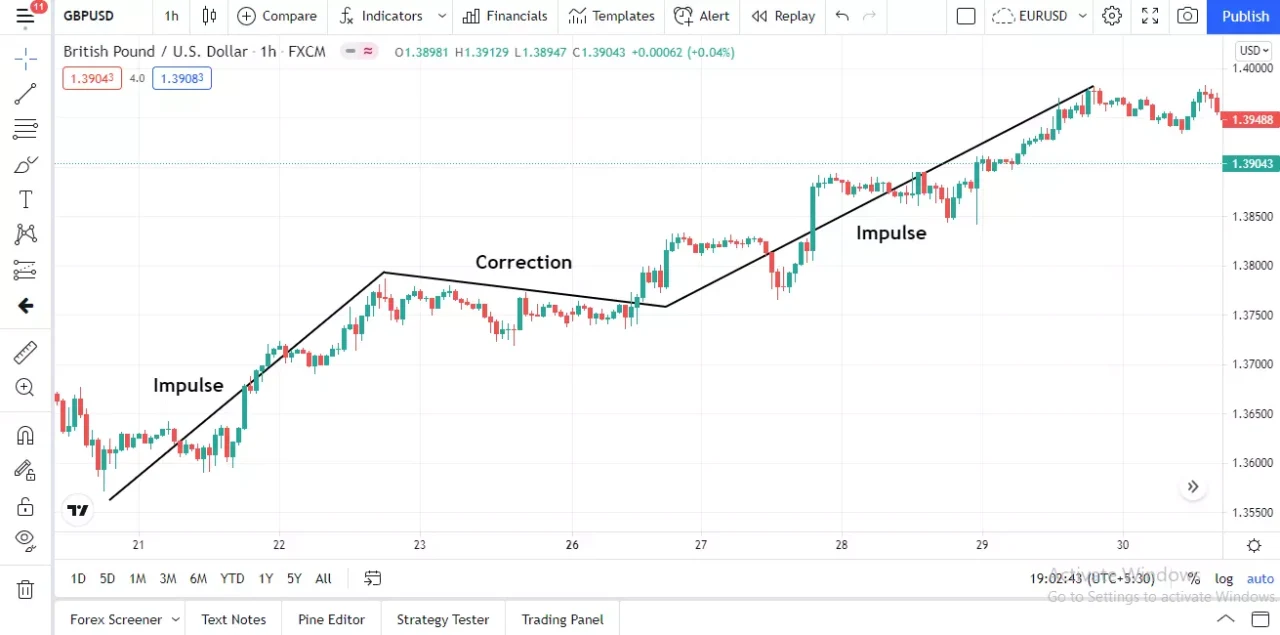The price of any financial asset does not move in a single direction all the time. It undergoes some phases, these phases are impulse and correction.

An impulse phase is when the price is trending in one particular direction with candlesticks of the appropriate color being printed each time.

It is impossible for the price to move in the same direction the entire time, hence there is always a correction phase.

A correction is when the price moves in the opposite direction to the trend or even moves in a sideways direction. This move is comparatively a minor move and is not considered to be a major trend being formed.

Corrections are also known as pullbacks or consolidation. In corrections, the candlesticks that are formed are often of mixed type and no particular candlestick being formed each time.

At any given time, price moves in these phases. After every impulse, there is a correction and after every correction, there is an impulse.

It is often noticed that after a correction, the next impulse move is in the direction of the previous impulse phase.

### 3. Plot the Fibonacci retracement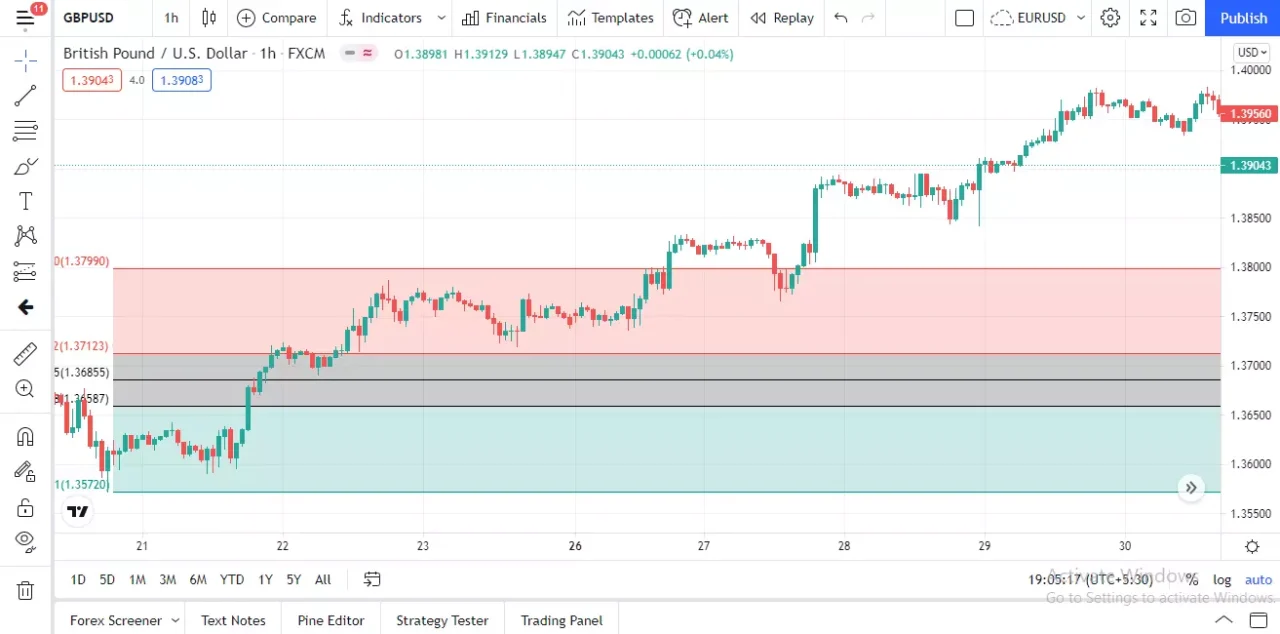Once you know the trend of the market, you know the direction in which the impulse move was headed. For this strategy, it is essential that you spot the correction phase of the price.

After spotting the impulse and correction, you need to find out when the correction will end i.e. exactly when will the price stop correcting and enter into a fresh impulse phase.

This is where the Fibonacci retracement tool comes into action.

The Fibonacci tool here is applied by plotting it across the swing highs and lows. In an uptrend, the tool is plotted by connecting the swing low to the swing high and in a downtrend, it is plotted by connecting the swing high to the swing low.

For the sake of this strategy, I suggest using only three ratios, 38.2%, 50%, and 61.8%. I believe that these ratios are the major ones and the remaining are not of much importance.

Once the Fibonacci retracement levels are plotted, you can expect the correction to end once it arrives near the three ratios. Taking a trade right when the price moves to these ratios is not a prudent thing to do.

It is always better to look for additional confirmations or confluences before a trade is taken. This will simply increase the probability of the trade being a successful one.

### 4. Find extra confirmations for entries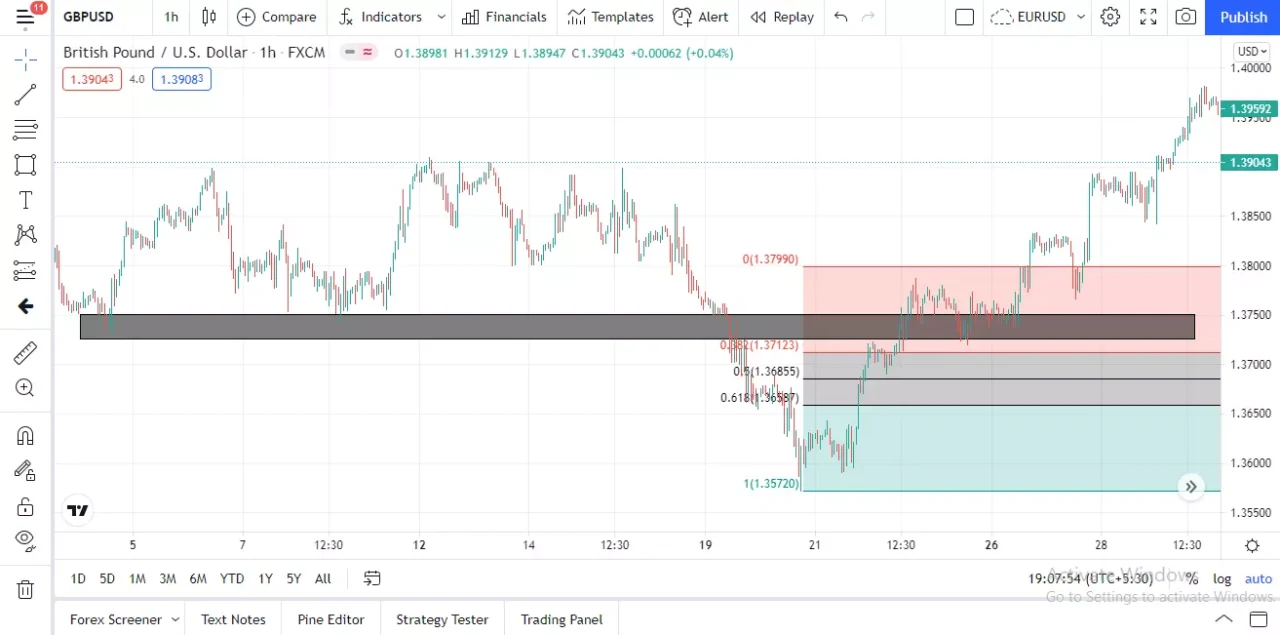Once the price of the financial asset arrives at the Fibonacci ratios, it can be seen as a possibility of a potential trade.

The price at such levels indicates that there might be a move that will take place and you must be attentive in order to enter the trade.

I’d suggest you look for certain candlestick patterns or even support and resistance levels around these Fibonacci retracement levels.

Before beginning the next impulse phase, there might be some candlestick patterns in the formation that traders can look for as a trigger to enter into the trades.

It is also noticed that the Fibonacci ratios are formed at the location as a support or resistance level that the price is respecting. This gives additional confirmation to the Fibonacci levels.

### 5. Stop loss and take profit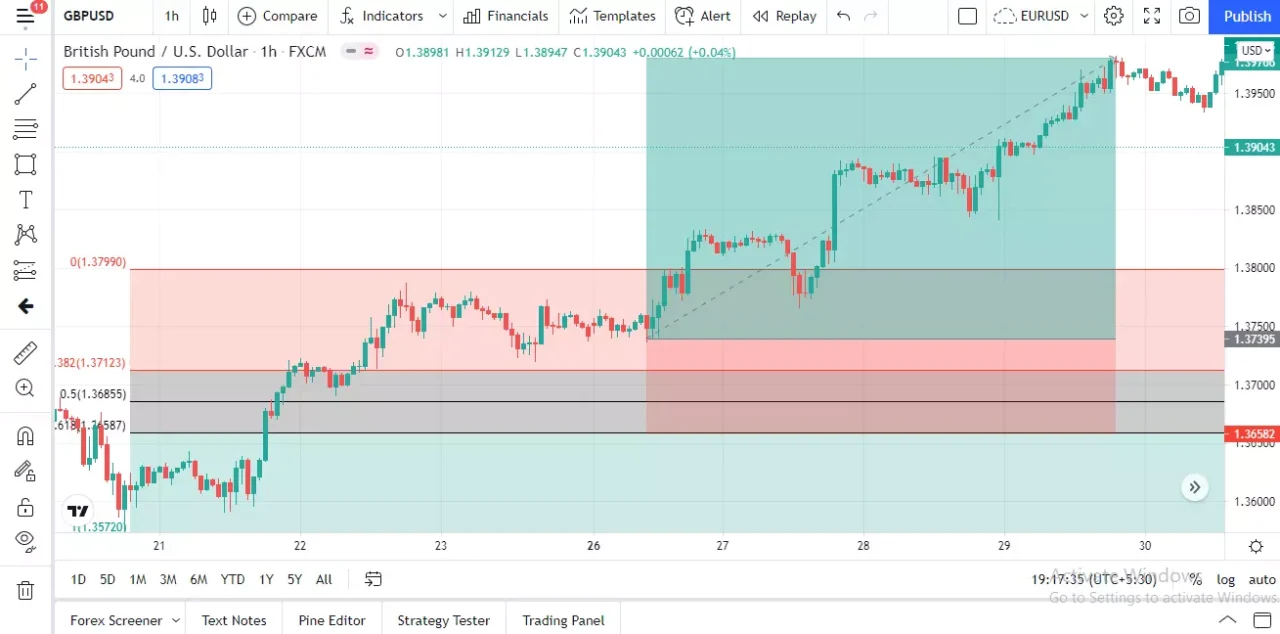After you have performed each step properly, it is time to set the stop loss and take profit for the trade.

With the help of the above-mentioned steps, you will get the entry price level for the trade, and the next important thing is the stop loss level.

There are many ways to place stop loss but in this strategy, the stop loss will be placed around the 61.8% level.

If the trade is a long one then the stop loss will be placed a few ticks or pips below the 61.8% level and if it is a short trade then the stop loss will be placed a few ticks or pips above the 61.8% level.

No matter at what point on the chart the trade was entered into i.e. either at the 38.2% or the 50% level, stop loss is to be placed around the 61.8% level only.

The take profit level in such trades is to be at the swing high or low. In a long trade take profit will be at the swing high and in a short trade, the take profit will be at the swing low.

The take profit plan can be altered a bit as it is not compulsory that the price will travel all the way to the desired level.

When I traded this strategy in the live markets, I had two methods for take profit, first either at the swing high or low, and the second could be to take profit at any nearby support and resistance.

I took partial profits at these levels in the trades by closing out a fraction of the position and allowing the remainder of the position to run in profits. This could also be one method that you can use.

Have you had any experience with the Fibonacci tools? How has it worked out for you? Do let me know.

One thing I want to point is that there is no guarantee that the strategy I have talked about in this blog post will have 100% results, in fact when I played out this strategy in the market I did have losing trades.

I would suggest you backtest this strategy on past data, understand what it seeks to achieve, learn the minute details and then take the decision whether to trade based on it or not.

Don’t forget to share this blog post with others and let them also take advantage of the Fibonacci retracement tool and they can earn profits too.

Feel free to reach out to me for absolutely anything through the comments section and I will get back to it at the earliest.